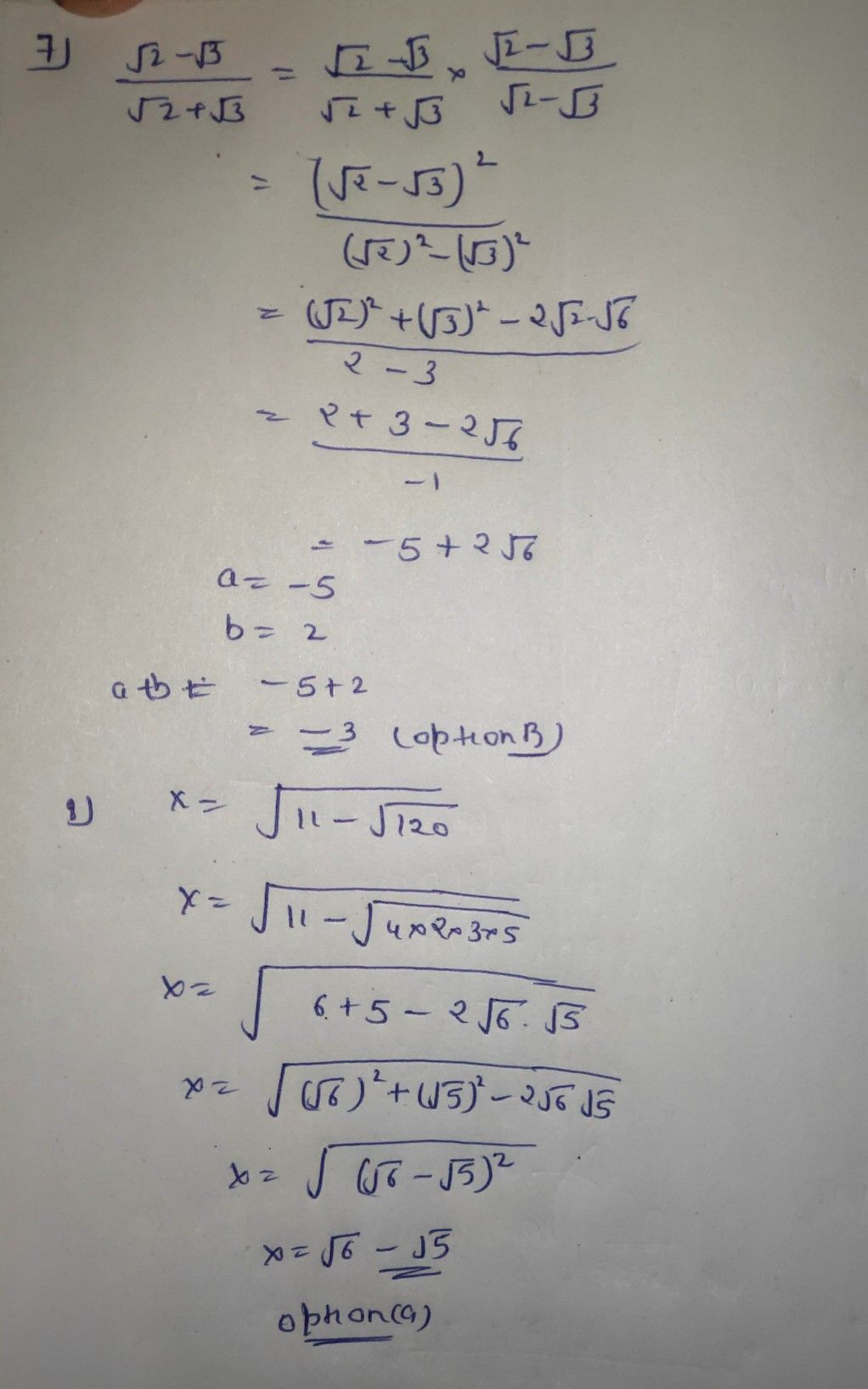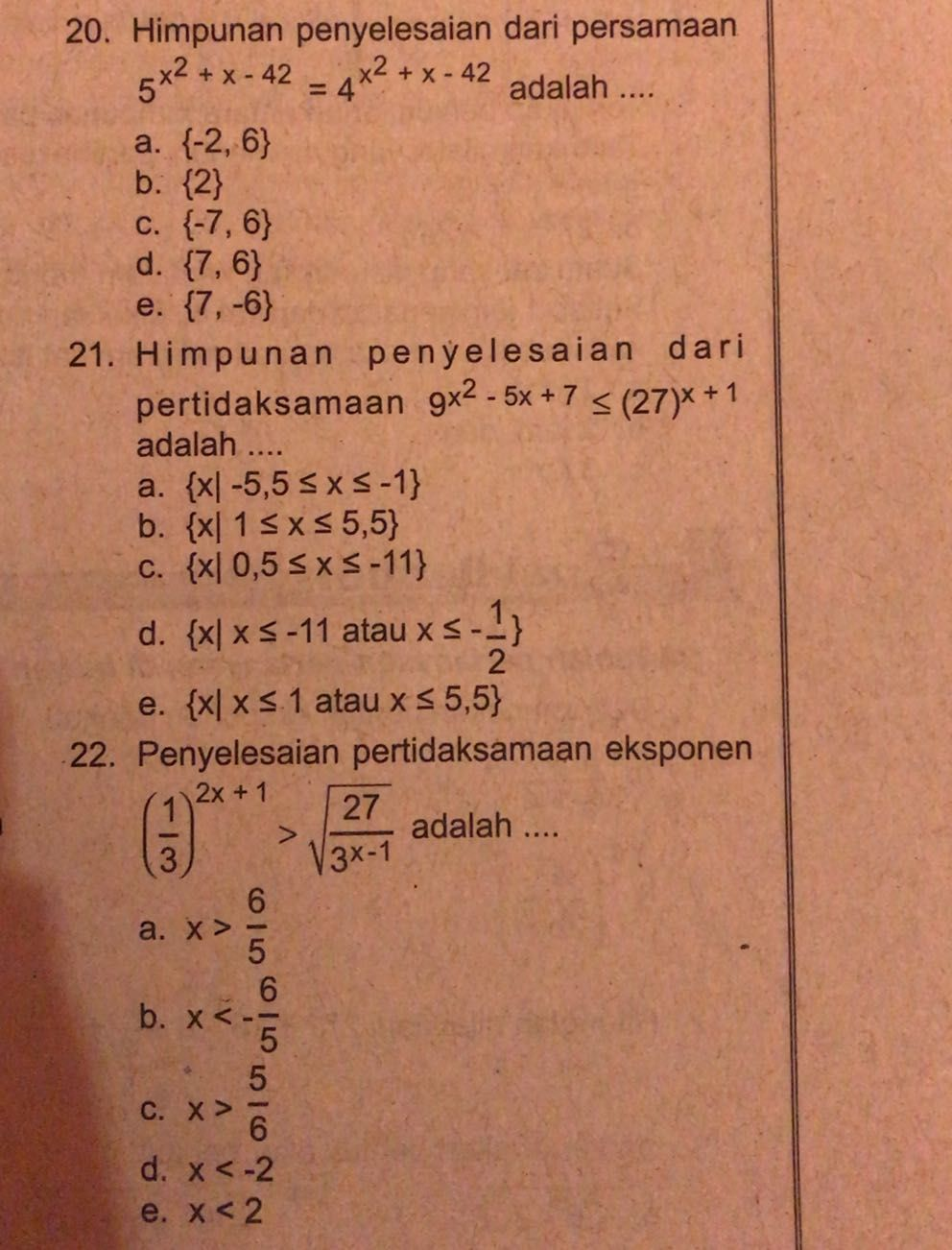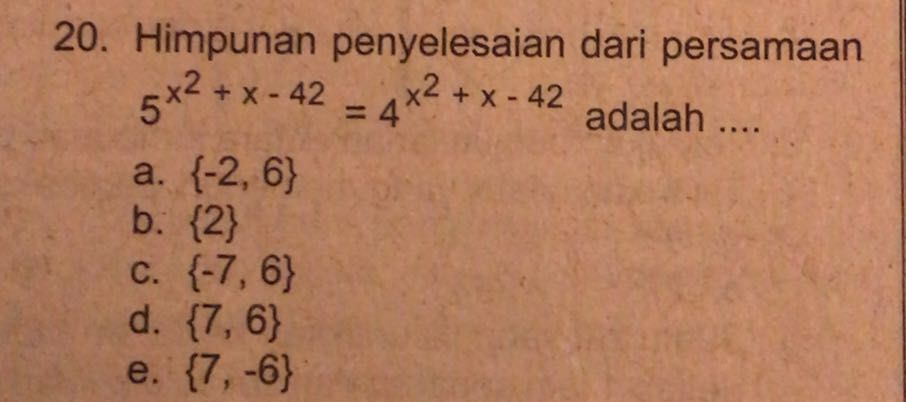Symbol
Problem$7.$ Jika $\dfrac {\sqrt{2} -\sqrt{3} } {\sqrt{2} +\sqrt{3} }.=a+b\sqrt{6}$ a dan b bilangan bulat, maka nilai dari $a+b$ adalah .. a. $-5$ d. $2$ b. $-3$ e. $3$ C. $-3$ $8.$ Bentuk sederhana dari $\sqrt{11-\sqrt{120} }$ adalah ... a. $\sqrt{6} -\sqrt{5}$ b. $\sqrt{6} +\sqrt{5}$ c. $\sqrt{11} -\sqrt{5}$ d. $\sqrt{11} +\sqrt{5}$ e. $\sqrt{15} -\sqrt{8}$
10th-13th grade
Other
Search count: 107
SolutionQanda teacher - AskhinaThanks for asking a question. You can ask any doubt without any hesitation in a direct way also for high-quality answers quickly and please rate 5 stars if you are satisfied with my answers And, if you have no doubt related to the previous question then please evaluate the answer and give some coins as a gift.Student
thank uQanda teacher - Askhina
welcomeStudent
may I ask you again?Qanda teacher - Askhina
yes
yes but dear plz understand to me only one question is allowed to give in one time (one chat room) by QandA...StudentQanda teacher - Askhina
u can ask again by preffered match
I think you may understand to me and qanda policyStudentQanda teacher - Askhina
I mean one question in one chat room